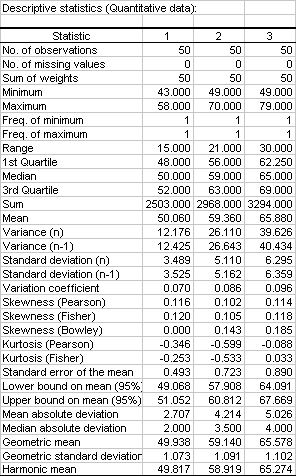# Box plot tutorial in Excel

2017-10-20

This tutorial shows how to draw and interpret box plots, also referred to as box and whisker plots in Excel using the XLSTAT software.

## Dataset for generating a box plot

An Excel sheet with both the data and the results can be downloaded by clicking on the button below:

The data correspond to a sample of 150 irises for which 4 variables were measured. The flowers belong to 3 different species. Fisher used this dataset, now famous, when he developed his discriminant analysis theory. In this particular example, we decided to analyze the variable Sepal length of the flowers and to visually check if there are differences between the three species using box plots (box-and-whisker plots).

The means ± symmetrical error bars representation is very common while only reliable if the data is normal. On the other hand box plots are adaptable to a broader range of distributions, as they are based on quantiles. Apart from letting you compare several distribution locations and widths with each other, boxplots let you check if the distribution is symmetrical or skewed, if there are outliers in the data, as well as the location of the mean within the distribution.

## Setting up the dialog box for the box plot

Once XLSTAT is open, select the XLSTAT / Visualizing data / Univariate plots command.Once you have clicked on the button, the Descriptive Statistics dialog box appears.

The data corresponding to the variable "Sepal length" were selected in the Quantitative data field. Note that for a box plot, the data must be numerical (quantitative) data.

As the name of the variable was included in the selection, the Labels included option was also selected.

The Species data were selected as subsamples to enable the comparison between species.

The Sheet option was selected because we wanted the results displayed on a new sheet of the workbook.In the Options tab, the following options have been activated.The Normalize or Rescale options can be used when you want to compare several variables spread over different scales - there is no need to use these in this case as we are dealing with only one variable.

The confidence interval does not play a role in generating boxplots, so we won't pay attention to this option.

In the Outputs tab, you can select different descriptive numerical statistics (mean, standard deviation, variance, skewness, kurtosis...) that will be computed for every subsample.

In the Charts(1) tab (charts related to quantitative data), and in the chart types subtab, check the box plots option.In the Options subtab, the Group plots option has been chosen so that the box plots are displayed on the same chart, and not separately.

The Minimum/Maximum option has been checked so that the minimums and maximums are represented on the box plots.

Notice that several display options are also available.Click on the OK button to obtain the results.

## Box plot interpretation

The results are displayed on the new sheet named "Desc". They include a full set of descriptive statistics.Then, the box plots are displayed.One box plot per species is displayed. The red crosses correspond to the means. The central horizontal bars are the medians. The lower and upper limits of the box are the first and third quartiles, respectively. Points above or below the the whiskers' upper and lower bounds may be considered as outliers. Points in blue are minimum and maximum for each species. The box plot's horizontal width has no statistical meaning.

It appears clearly that the Sepal length variable is different (higher) for the third species compared to the first two.

Watch this video to see how to generate this boxplot.

#### Contact our technical support team: support@xlstat.com

https://cdn.desk.com/
false
desk